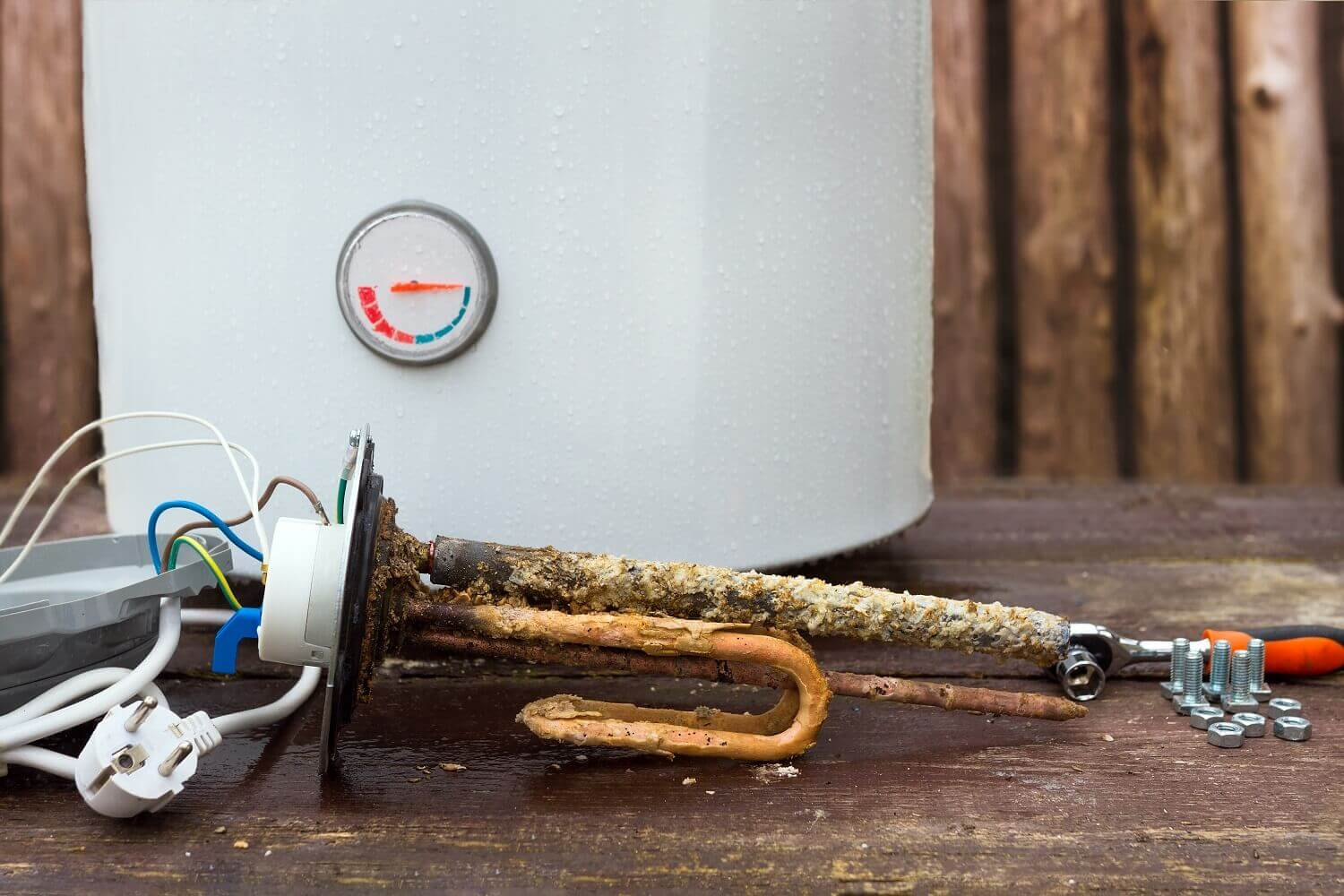# Identifying The Age Of Your Water Heater: Here's How To DoThis article is an easy reference guide to the most common producers of water heaters.  Regrettably, many producers of water heaters do not explicitly specify the manufacturing year on the data label or plate. Still, fortunately, the year of production is often included within the serial number. This article discusses how to identify the age of your water heater.

## Water HeatersUSAAO Smith

Y= Year of Manufacture X= Letter 7=Numbers

The production date for AO Smith water heaters gets recorded in serial numbers.

YY77X7777777 (First and Second digits of serial numbers refer to the year of production)

YY777 77777 (First and Second digits of serial numbers refer to the year of production)

YY77777777777 (First and Second digits of serial numbers refer to the year of production)

Note: Old heaters might hold added alphabets preceding the first and second digits

## Apollo

Y=Year of Manufacture X= Letter 7= Numbers

The year of Apollo water heaters' production is recorded within the serial numbers.

XYYX77777 (First and Second digits of serial numbers refer to the year of production)

Y= Year of Manufacture X= Letter 7= Numbers

The year of production for Bradford White water heaters gets recorded within the serial numbers.

YX7777777 (The first alphabet of the serial numbers refers to the year of production.)

A=2004 B=2005 C=2006 D=2007 E=2008 F=2009 G=1990/2010 H=1991/2011 J=1992/2012 K=1993/2013  L=1994/2014

M=1995/2015 N=1996/2016 P=1997/2017 S=1998/2018 T=1999/2019 W=2000/2020 X=2001/2021 Y=2002/2022 Z=2003/2023

## Craftmaster

Y=Year of Manufacture X= Letter 7= Numbers

The year of production for Craft master water heaters is recorded in their serial numbers.

YY7777777 (First and Second digits of serial numbers refer to the year of production)

## General Electric (GE)

Y= Year of Manufacture X= Letter 7= Numbers

The year of production for General Electric water heaters gets recorded within the serial numbers.

77YY000000 (Third and Fourth digits of serial numbers refer to the year of production)

XX77YY777777 (Third and Fourth digits of serial numbers refer to the year of production)

Gas models:  GENG 77YYX77777 (Third and Fourth digits of serial numbers refer to the year of production)

## Navien

Y=Year of Manufacture X= Letter 7= Numbers

The year of production for Navien water heaters gets recorded within the serial numbers.

7777XYY77777777 (Fifth and Sixth digits following an alphabet of serial numbers refer to the year of production)

7777XYYX7777777 (Fifth and Sixth digits following an alphabet of serial numbers refer to the year of production)

7777-YYYY7777-7777 (Fifth, Sixth, Seventh, and Eighth digits of serial numbers refer to the year of production)

7777-YYYY-777-7777 (Fifth, Sixth, Seventh, and Eighth digits of serial numbers refer to the year of production)

## Rheem/Ruud

Y= Year of Manufacture X= Letter 7= Numbers

The year of production for Rheem/Ruud water heater is in serial numbers.

X77YYX7777777777X (Third and Fourth digits following an alphabet of serial numbers refer to the year of production)

Tankless   YY.77-777777 (First and Second digits after an alphabet of serial numbers refer to the year of production)

## Rinnai

Y= Year of Manufacture X= Letter 7= Numbers

The year of production for Rinnai water heaters is in serial numbers.

2009 or eventually Y7. CA-777777 (The first alphabet of serial numbers refers to the year of production)

A=2009 B=2010 C=2011 D=2012 E=2013 F=2014 G=2015 H=2016 J=2017 K=2018 L=2019 M=2020 N=2021 P=2022 Q=2023

Preceding 2009    YY. 77-77777 (First and Second digits of serial numbers refer to the year of production)